# More chip counting exercises

If our first batch of chip counting exercises was no problem, here’s five more to test your chip-counting skills! Are you good enough at counting chips to quickly calculate the correct totals?

You can click the images to zoom in. Each stack shown contains the same number of chips as the stack splashed next to it. The chips use the standard denominations:

• Yellow—50¢
• White—\$1
• Red—\$5
• Green—\$25
• Black—\$100
• Purple—\$500

This time, we’re including some exercises using a chip rack. Each row of chips shown in the rack represents a full tube of 20 chips. Don’t forget to include these in your total!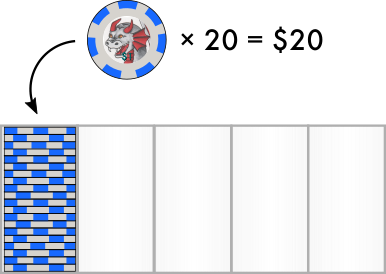## Exercises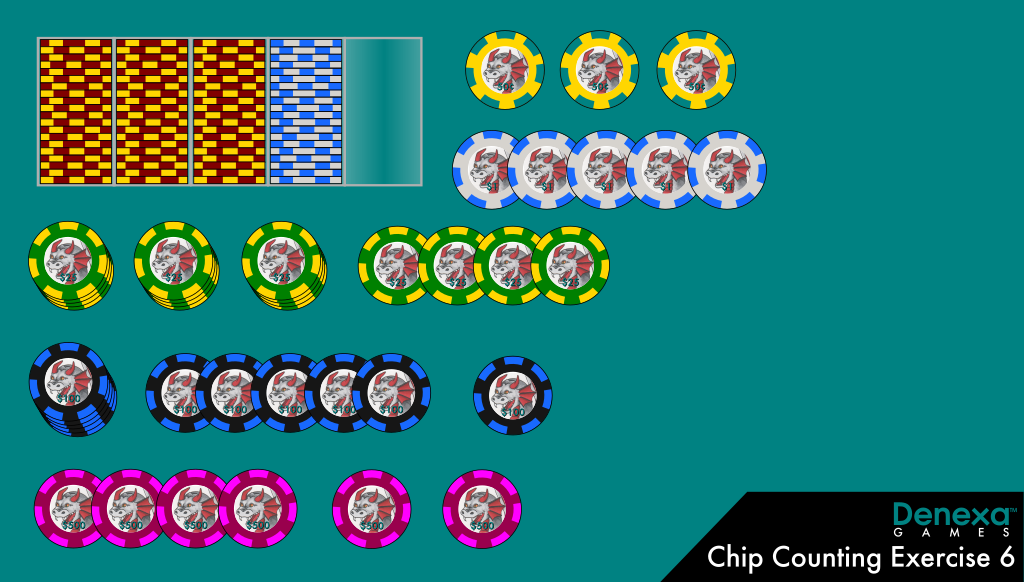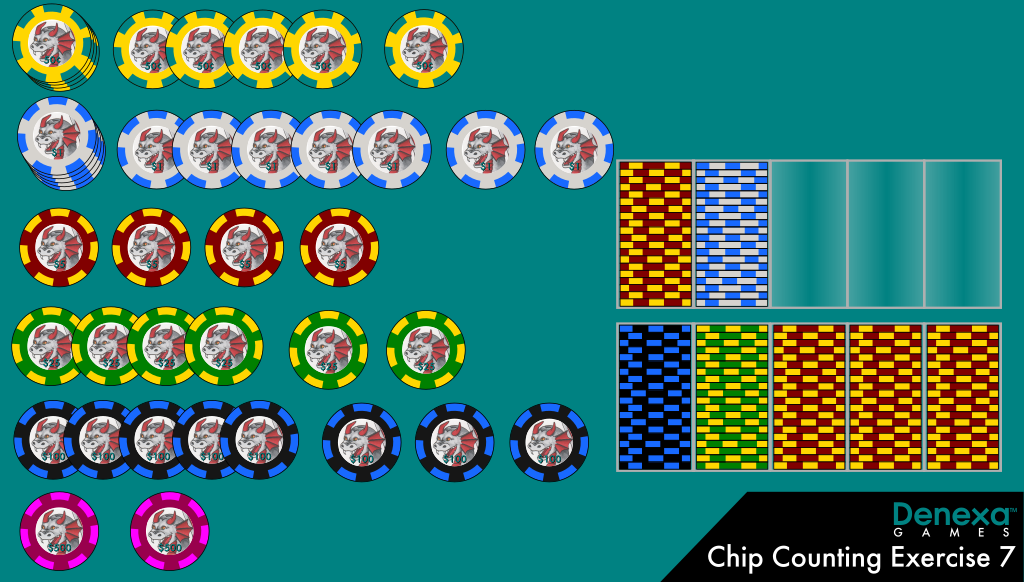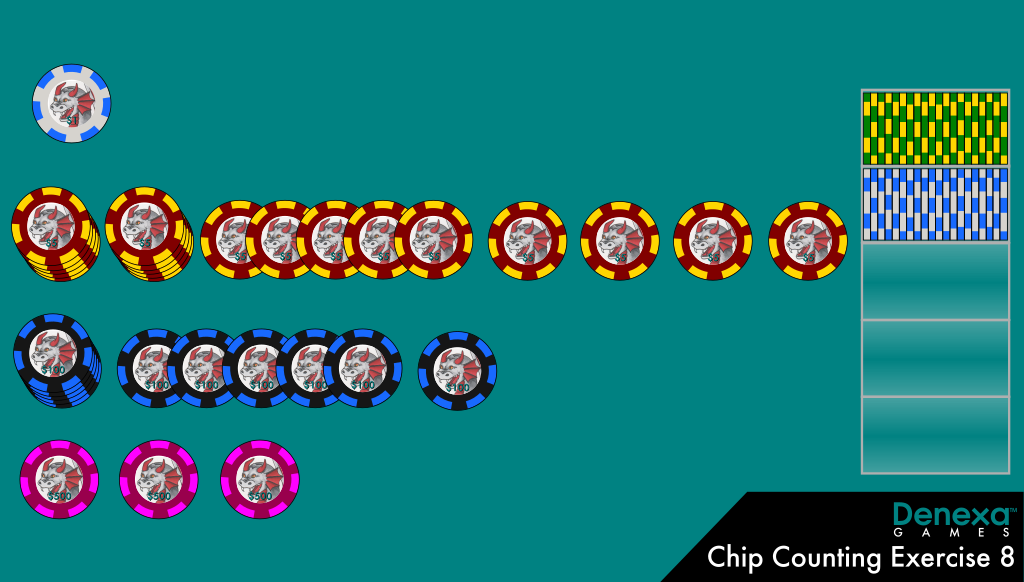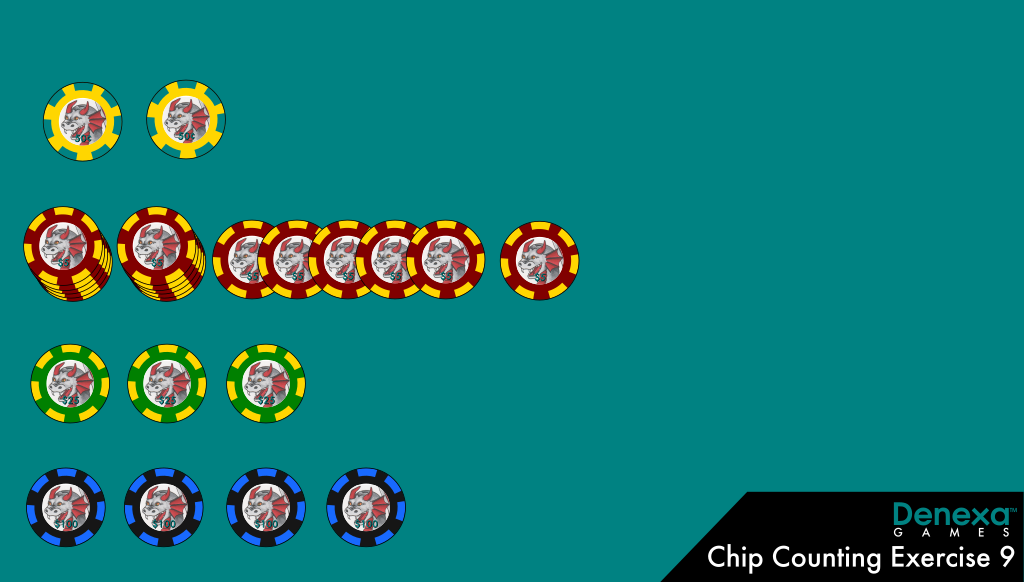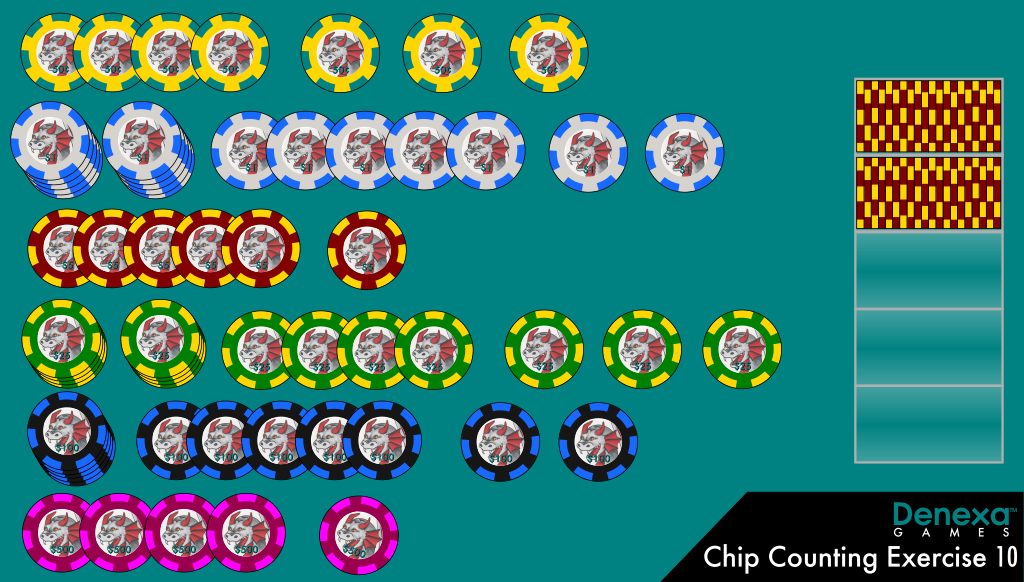1. 6 × 500 = 3000, 11 × 100 = 1100, 16 × 25 = 400, 60 × 5 = 300, 25 × 1 = 25, 3 × 50¢ = 1.50. 3000 + 1100 + 400 + 300 + 25 + 1.50 = 4826.50.
2. 2 × 500 = 1000, 28 × 100 = 2800, 26 × 25 = 650, 84 × 5 = 420, 32 × 1 = 32, 9 × 50¢ = 4.50. 1000 + 2800 + 650 + 420 + 32 + 4.50 = 4906.50.
3. 3 × 500 = 1500, 11 × 100 = 1100, 20 × 25 = 500, 19 × 5 = 95, 21 × 1 = 21. 1500 + 1100 + 500 + 95 + 21 = 3216.
4. 4 × 100 = 400, 3 × 25 = 75, 16 × 5 = 80, 2 × 50¢ = 1. 400 + 75 + 80 + 1 = 556.
5. 5 × 500 = 2500, 12 × 100 = 1200, 15 × 25 = 375, 46 × 5 = 230, 17 × 1 = 17, 7 × 50¢ = 3.50. 2500 + 1200 + 375 + 230 + 17 + 3.50 = 4325.50.

### 2 responses to “More chip counting exercises”

1.TDiddlez says:

Exercise 7…I only see 32 dollar chips? for a total of 4906.50

2.Denexa Games says:

You’re absolutely right! We’ve fixed that. Nice catch! (Ever thought about becoming a cashier? You’d have saved the casino \$4! 😛 )